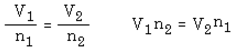# Avogadro’s Law Formula

Provides the association amongst amount and volume when temperature and pressure are held at standard. The amount is measured in moles, always remember that. Similarly, the container holding the gas is flexible in some way and can expand or contract because the volume is one of the variables.

If the amount of gas in a container is amplified, the volume upsurges.

If the quantity of gas in a container is reduced, the volume declines.

Why?

Let’s assume that the quantity is greater than before. This means that there are numerous gas molecules, and this will intensify the number of impacts on the vessels walls. This can be comprehended as the gas pressure inside the vessel will grow (instantaneously), reaching a pressure greater than on the exterior of the walls. Thus, the walls move outwards. As there is added wall space, and the pressure will return to its original value as the impacts will lessen.

The scientific form of Avogadro’s Law is:

V ÷ n = k

This means that the volume amount fraction will always be the same value if the pressure and temperature remain constant.

Let V1 and n1 be a volume amount pair of data at the commencement of an research. If the amount is transformed to a different value called n2, then the volume will b altered to V2.

As we are aware that  V1 ÷ n1 = k

And we are acquainted with: V2 ÷ n2 = k

Meanwhile as k = k, we can determine that V1 ÷ n1 = V2 ÷ n2.

This equation of V1 ÷ n1 = V2 ÷ n2 will be very useful in cracking Avogadro’s Law problems. Here is the Law articulated in fractional form:The Avogadro’s Law is a direct mathematical relationship.

Explained Samples

Problem 1: What volume of O2 measured at 1.86 ×× 106Pa and 375k, is required to react entirely with 1.78l of H2, measured at the same temperature and pressure, to give H2O?

Initially, create a stable equation which is balanced for the reaction.

2H2   +   O2 →→ 2H2O

2mole  1mole

One gets to know from the equation that the molar amount of H2 is double the molar amount of O2 needed. By avogadro’s law, at the constant temperature and pressure n is proportional to V. Therefore, the volume of O2 needed is

Note that the temperature and pressure values stated in the problem were not required in the calculation.

Problem 2: How many molecules of O2 are present in 1.00L of O2 at STP?